# SN Dey Class 11 Solutions Compound Angles Very Short Answer Type Questions

SN Dey Class 11 Chapter  Solutions. Compound Angles VSATQ. Solutions of Trigonometric Ratios of Compound Angles Very Short Answer Type Questions

Ex 1(i):  Find the Value of

sin(-75°)

Solution: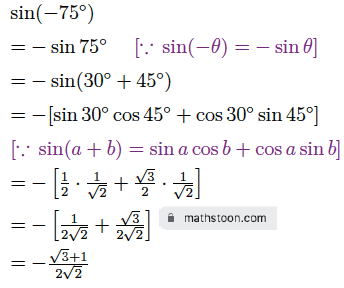Ex 1(ii):  Find the Value of

cos15°

Solution: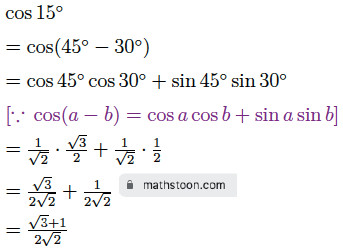Ex 1(iii):  Find the Value of

tan(-105°)

Solution: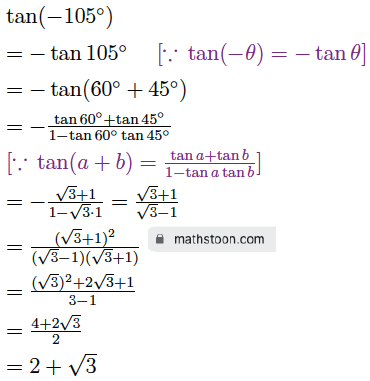Ex 1(iv):  Find the Value of

sec(-75°)

Solution: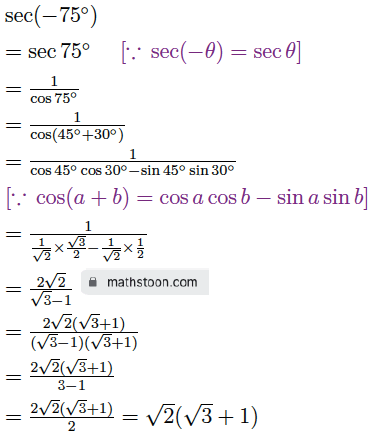Ex 2(i):  Prove that tan75° – cot75° = 4 sin60°

Solution: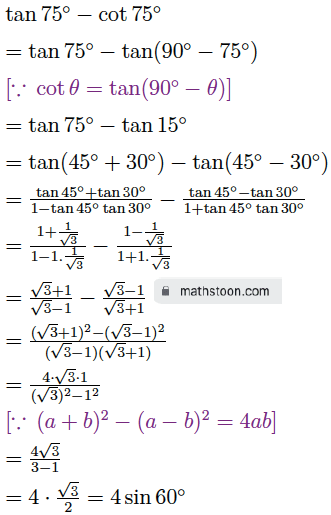Ex 2(ii):  Prove that cosα + cos(120°+α) + cos(120°-α) = 0

Solution: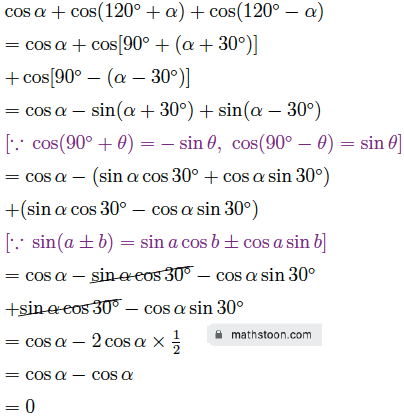Ex 2(iii):  Prove that tan35° + tan10° + tan35° tan10° = 1

Solution: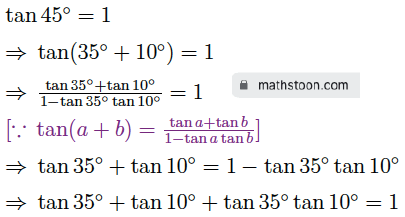Ex 2(iv):  Prove that tan8α – tan5α – tan3α = tan8α tan5α tan3α

Solution: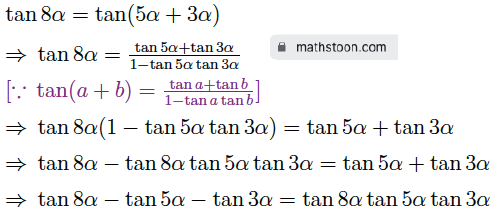Ex 2(v):  Prove that tan43° = $\dfrac{\cos 2^\circ-\sin 2^\circ}{\cos 2^\circ+\sin 2^\circ}$

Solution: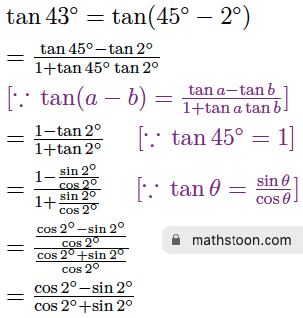Ex 2(vi):  Prove that tan62° = 2 tan34° + tan28°

Solution: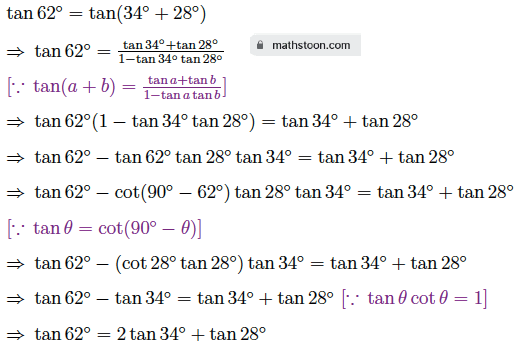Share via: